##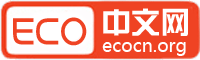帐号 自动登录 找回密码 密码 立即注册
 搜索
ECO中文网 门户 优秀译文推荐 博客 查看内容

# [2011.10.04] 2011年诺贝尔物理学奖

2011-10-5 10:21| 发布者: Somers| 查看: 3976| 评论: 12

 2011年诺贝尔物理学奖给我们开阔了视野Oct 4th 2011, 12:39 by G.C.毫不夸张地说，今年的诺贝尔奖物理学授予了有史以来最重大的物理学发现——宇宙不仅在扩张（这20世纪20年代以来已为世人所知），而且扩张的速度越来越快。换种说法，某种力量正在活力十足地使它四散膨胀。这一结论是20世纪90年代两个小组分别发现的。他们当时在研究被称作超新星的爆炸恒星。一个是萨尔•波尔马特领衔的加州大学伯克利分校的超新星宇宙学计划小组。另一个是布莱恩•施密特主持、亚当•里斯参与的国际项目高红移超新星搜寻队，当时他们两人都供职于哈佛大学。他们三位将分享诺贝尔物理学奖。超新星有各种不同类型。不过，有一类被称为Ia型的超新星爆炸时释放的能量始终基本相同，因此量度也相同。这意味着可以利用它们相当精确估计它们的距离（因而可以估计他们所在的星系的距离）。此外，因为宇宙在膨胀，所以可以通过其红移计算出一个正在远离地球而去的物体（如恒星或星系）的速度。红移是指物体光波频率下降，在光谱上出现在红端。这是由多普勒效应（类似一辆警车或消防车鸣着警笛从你身边经过，之后音高突然下降）引起的。两个小组均发现，遥远的超新星发出的光比预期的暗淡。换句话说，基于现有的宇宙模型，超新星的实际距离要比其红移显示的距离远。这说明有某种力量正在使宇宙变大。到底是什么东西，目前只能猜想。它被标为“暗能量”，但这其实是物理学家们的简略表达方式，因为“我们还没有一点头绪”。不过，它可能涉及到一个叫做宇宙常数的数学术语，它在爱因斯坦的广义相对论里面出现过。在宇宙膨胀现象发现之前，爱因斯坦认为该常数对阻止宇宙崩塌不可或缺。     感谢译者 tribesman 点击此处阅读双语版

### 最新评论

0o。 2011-10-5 00:43
is a fall in the frequency of its light towards the red end of the spectrum、

tribesman 2011-10-5 07:49

Thanks.

1. in a sense literally的理解，我也不太确定，求高人指导啊~
2.It is these three gentlemen who have shared the prize.“他们三位将分享诺贝尔物理学奖”

It is these three gentlemen who have shared the prize.

This was worked out by two groups who, in the 1990s, were studying exploding stars called supernovae.

VIP子非鱼 2011-10-5 21:02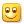很感谢，希望对于我今年的考研有帮助
fctfct 2011-10-5 22:11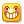tribesman 2011-10-6 09:19

afans 2011-10-8 09:14

allenliou 2011-10-13 00:14
It is surprised to hear that the three U.S. physicists had won the Nobel Prize in physics for discovering through the study of supernovae that the universe is accelerating expansion.
The Hubble’s redshift and the Doppler Effect are the facts. It doesn’t mean the universe is expansion or universe is accelerating expansion.
The expansion of the universe is based on the true of the Big Bang theory.  If the Big Bang theory is not true. Even though the Hubble’s redshift, and the Doppler Effect is the facts. The expansion or accelerating expansion of the universe is not supported.
The Big Bang theory and “dark energy” are not just a crazy idea, it is nonsense. Is anyone believe the “dark energy” able to create the real energy to push the supernovae accelerating expansion? If you do believe it. You may believe “dark human” can turn spirit to a real person.
In Scientific community , the Big Bang and ”dark energy” issue  had been argue for so many year, And now act rashly to award  the Nobel Prize in physics for the universe is accelerating expansion .I believe that someday will be proved the 2011 of the Nobel Prize for physics turn out to be a joke.
The expansion of Universe should not true. If it is true, the Big Bang theory and “dark energy” will be true.
Now raise a big question. If he Big Bang theory and “dark energy” are not true. Also the Hubble redshift, and the Doppler Effect is the facts, How could be proved the universe is not expansion or not accelerating expansion?
The space of the universe has only three kind of possibility. One is Euclidean space, one is elliptical space and the other is hyperbolic space. These three kinds of space can only be hypnosis to be one of the three only, And, it cannot be identify by proved.
1.        If the universe is a Euclidean space, due to the Hubble redshift, and the facts of the Doppler Effect, There must be the expansion of the universe and the Big Bang also true. This is contradiction to the Big Bang is not true. So, the space of  the   universe may not be a Euclidean space
2.        The space of the universe will never be an Elliptical space. If the space of the Universe is an Elliptical space. When we see anything from the east, can be seen from the west too. Obviously, it is not so
3.        Remaining space is The Hyperbolic space.

Let analyze, if supernovae happen in Hyperbolic space.

Hubble’s laws are derived from Euclidean rules and Euclidean formulas. However, assume the Universe is in Hyperbolic space. Very logically, we must derive its rules and formulas from Hyperbolic rules and non-Euclidean formulas.

The rules and formulas of Hyperbolic space are quite different from Euclidean space. Hence, the results derived from utilizing these two systems must be different. These differences may be the keys to unveil the mystery of the Universe.
“Now we try to prove, the universe is not expansion or not accelerating expansion. Even though Hubble’s redshift and the Doppler effect are the facts”

A. LIGHT SPHERICAL WAVE FRONTS

When photon travel a distance of r. The equation of a light spherical front in Euclidean space is

x^2 + y^2 + z^2 =  r^2          --------------   (1)

From Hyperbolic geometry, the equation of the light spherical front is

tanh^2 x/k  + tanh^2 y/k  + tanh^2 z/k  = tanh^2 r/k   ------ (2)

Where k is the constant of the space curvature. （cosmological constant）
(From page 298 of non-Euclidean Geometry by Allen Liou, 1964.)

Comparing equations (1) and (2), we can see very obviously that the area of the light spherical fronts is very much different. Even though they have the same radius. Therefore, the Doppler Effect should not be the same between Euclidean space and Hyperbolic space.

The area of the Light Spherical Front in Euclidean space is 4πr^2.
What is the area of the Light Spherical Front in Hyperbolic space?

Let us determine the circumference of a circle in Hyperbolic space first:

Let PQ be the chord of a circle of radius r, which subtends an angleθ, M be the midpoint of the chord, and O be the center of the circle.
See pic 1.

From the formula of the right-angle in Hyperbolic trigonometry, we have (page 143 of non-Euclidean Geometry by Allen Liou, 1964.)

sinh PQ/2k  = sinh r/k sin∠POQ/2

If  angle θ 0
We have                    ds/2k  = sinh r/k dθ/2
or           ds  = k sinh r/k dθ

Integrating both sides, we have

Circumference  = 2πk sinh r/k

Then, let ds are the length of the arc of the spherical circle, and r  be the radius.
By same formula, we have, see pic 2.

ds  = k sinh r/k dθ

The area of the circle strip is
d (area of circle strip) = 2πk sinh AM/k ds

But
sinh AM/k  =  sinh r/k sinθ

Therefore
d(area of circle strip)=2πk[sinh r/k sinθ][k sinh r/k dθ]
= 2πk^2 sinh^2 r/k dθ

Integrating both sides, we have
area of sphere  =  4πk^2 sinh^2 r/k

B. DOPPLER EFFECT OR “LIOU’S STRETCH EFFECT”

When a photon travels a distance r, the area of the Light Spherical Front in Euclidean space is 4πr2.

area of sphere  =  4πr^2

But the area of the Light Spherical Front in Hperbolic space is

area of sphere  =  4πk^2 sinh^2 r/k

Compare the two Spherical Areas in the two different spaces with the same r. We easily to see that, if we are in Hyperbolic universe, Light Spherical Front stretch from 4πr 2 to 4πk 2 sinh2 r/k. We temporary called this stretch by “Liou’s stretch effect”.

The Spherical Front of photon may only travel a distance r in Hyperbolic space. But in Euclidean space, it appears to travel a distance of k sinh r/k.

From the difference of r and k sinh r/k in Euclidean space, it looks like the object moves from point r  to point k sinh r/k, but the object actually stays still in Hyperbolic universe.

When we use Redshift of Doppler Effect in Euclidean space to calculate velocity of galaxy from point r  to point k sinh r/k. Actually,  there is no movement from point r  to point k sinh r/k. It only cause by the stretch of curvature of Hyperbolic space (“Liou’s stretch effect”).

I will use the velocity to calculate the space constant (cosmological constant). Use redshift of frequency the result is the same.

D. CALCULATION OF SPACE CURVATURE (OR COSMOLOGICAL CONSTANT) IN HYPERBOLIC UNIVERSE

Let                            s  = k sinh r/k - r

Where s is the distant of galaxy moving from position r  to the position k sinh r/k.
Taking the derivative of both sides, we have

ds/dt  = cosh r/k dr/dt - dr/dt

where ds/dt =v (the velocity of galaxies at the remote distance of r), and dr/dt is the speed of light c.

Therefore
v  = cosh r/k c – c

v  = c[cosh r/k - 1] = 2c sinh^2 r/2k ---------- (3)

There are several versions of the Hubble's constant. We will select the one most popular one. In which, the velocity of galaxies at a distance of six billion light-years move away at a velocity of roughly 90,000 kilometers/sec.
Hence v=90,000 kilometers/sec and r=6 bly.

Hence we have

v = 90,000 kilometers/sec and r = 6 bly.

Therefore
90,000   =  2c sinh^2（6bly/2k ）

∴  0.3c = 2c sinh^2（3bly/k ）

∴  k = 3bly/ sinh-1√0.12

∴  k = 7.931965828 bly

Where bly is billion light-years.

C. DISCUSIONS

1. Hubble’s constant was not constant.

From equation (3), v = 2c sinh^2 r/2k , the velocity of galaxies and the remote distance of r were not exactly linear proportions in Euclidean universe. The velocity is more likely in slightly acceleration observed in Euclidean universe.

Here, the cosmological constant, k = 7.931965828 bly was based on the Hubble’s Law at 6 bly. If we based on a difference distance, like one on a distance of 1 or 2 bly, the k value should be slightly different. If we use different versions of Hubble’s Law, the cosmological constant k will be even more different. We really need an accurate data to determine the constant k

Assuming k = 7.931965828 bly is correct; the Hubble's diagram in Euclidean space should look like the following diagram.

From this chart, we should call Hubble’s accelerator instead of Hubble’s constant.

2. Is Universe’s redshift cause by DOPPLER EFFECT or “LIOU’S STRETCH EFFECT”?

From Hubble’s Law, the speed by which a galaxy moves away is proportional to the distance to the galaxy.  A galaxy with distance of 6 bly has a velocity of 90,000 km/s. For a galaxy 30 bly away, its speed will be 450,000  km/s. This is beyond the speed of light a lot. It is contradict to the fact of the speed of light is constant.
In recent year, astronomer's observed that Hubble’s constant is not constant. The galaxies actually moving away accelerated, like supernovae.

From this two facts, the Universe’s redshift is more likely to be caused by the “LIOU’S STRETCH EFFECT”.
. And Doppler Effect caused by the STRETCH of the Hyperbolic space (“LIOU’S STRETCH EFFECT”) not by the speed of velocity in Euclidean space.

So, the universe is not expansion nor accelerating expansion. Even though Hubble’s redshift and the Doppler effect are the facts.

In other words,The universe is still in Hyperbolic space
handsome2011 2011-11-27 20:25

## 相关分类

need to be deleted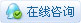|小黑屋|手机版|网站地图|关于我们|七月天| ECO中文网 ( 京ICP备06039041号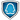GMT+8, 2022-5-19 14:05 , Processed in 0.070475 second(s), 27 queries .